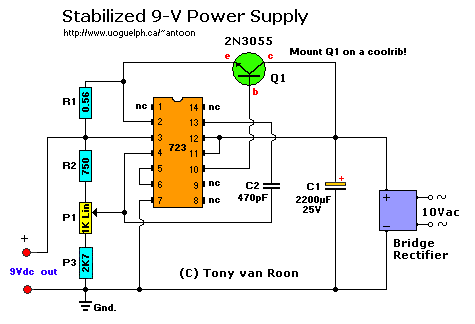```Parts List:
T1 = 115/10 VAC transformer. Center Tap (ct) not needed.
IC1 = uA723, LM723, or equivalent.
Q1 = 2N3055, NTE130, or substitute. (TO-3 case) Mount on a coolrib!
BR1 = 80V-5A (or better)
R1 = 0.56 Ohm, 1 Watt, wire-wound type, 5%
R2 = 750 Ohm, 5%
R3 = 2K7 (2700 ohm)
P1 = potentiometer, 1K, Linear
C1 = 2200uF, 35V
C2 = 470pF